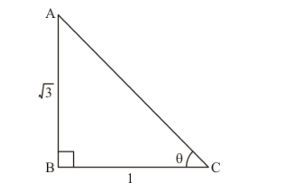# If cot θ=13√, show that 1−cos2 θ2−sin2 θ=35.

Question:

If $\cot \theta=\frac{1}{\sqrt{3}}$, show that $\frac{1-\cos ^{2} \theta}{2-\sin ^{2} \theta}=\frac{3}{5}$.

Solution:

Given: $\cot \theta=\frac{1}{\sqrt{3}}$....(1)

To show that $\frac{1-\cos ^{2} \theta}{2-\sin ^{2} \theta}=\frac{3}{5}$

Now, we know that $\cot \theta=\frac{1}{\tan \theta}$

Since $\tan \theta=\frac{\text { Perpendicular side opposite to } \angle \theta}{\text { Base } \text { side } \text { adjacent to } \angle \theta}$

Therefore,

$\cot \theta=\frac{1}{\frac{\text { Perpendicular side opposite to } \angle \theta}{\text { Base side adjacent to } \angle \theta}}$

Therefore,

$\cot \theta=\frac{\text { Base side adjacent to } \angle \theta}{\text { Perpendicular side opposite to } \angle \theta}$....(2)

Comparing Equation (1) and (2)

We get,

Base side adjacent to $\angle \theta=1$

Perpendicular side opposite to $\angle \theta=\sqrt{3}$

Therefore, Triangle representing angle $\theta$ is as shown belowHypotenuse AC is unknown and it can be found by using Pythagoras theorem

Therefore by applying Pythagoras theorem

We get,

$A C^{2}=A B^{2}+B C^{2}$

Therefore by substituting the values of known sides

We get,

$A C^{2}=(\sqrt{3})^{2}+1^{2}$

Therefore,

$A C^{2}=3+1$

$A C^{2}=4$

$A C=\sqrt{4}$

Therefore,

$A C=2$...(3)

Now, we know that

$\sin \theta=\frac{\text { Perpendicular side opposite to } \angle \theta}{\text { Hypotenuse }}$

Now from figure (a)

We get,

$\sin \theta=\frac{A B}{A C}$

Therefore from figure (a) and equation (3) ,

$\sin \theta=\frac{\sqrt{3}}{2}$....(4)

Now, we know that

$\cos \theta=\frac{\text { Base side adjacent to } \angle \theta}{\text { Hypotenuse }}$

Now from figure (a)

We get,

$\cos \theta=\frac{B C}{A C}$

Therefore from figure $(a)$ and equation $(3)$,

$\cos \theta=\frac{1}{2}$...(5)

Now, L.H.S of the equation to be proved is as follows

L.H.S $=\frac{1-\cos ^{2} \theta}{2-\sin ^{2} \theta}$

Substituting the value of $\sin \theta$ and $\cos \theta$ from equation (4) and (5)

We get,

L.H.S $=\frac{1-\left(\frac{1}{2}\right)^{2}}{2-\left(\frac{\sqrt{3}}{2}\right)^{2}}$

L.H.S $=\frac{1-\frac{(1)^{2}}{(2)^{2}}}{2-\frac{(\sqrt{3})^{2}}{(2)^{2}}}$

L.H.S $=\frac{1-\frac{1}{4}}{2-\frac{3}{4}}$

Now by taking L.C.M. in numerator as well as denominator

We get,

L.H.S $=\frac{\frac{(4 \times 1)-1}{4}}{\frac{(4 \times 2)-3}{4}}$'

Therefore,

$L . H . S=\frac{\frac{4-1}{4}}{\frac{8-3}{4}}$

$L . H . S=\frac{\frac{3}{4}}{\frac{5}{4}}$

Therefore,

L.H.S $=\frac{3}{4} \times \frac{4}{5}$

$L . H . S=\frac{3}{5}=R . H . S$

Therefore,

$\frac{1-\cos ^{2} \theta}{2-\sin ^{2} \theta}=\frac{3}{5}$

Hence proved that $\frac{1-\cos ^{2} \theta}{2-\sin ^{2} \theta}=\frac{3}{5}$